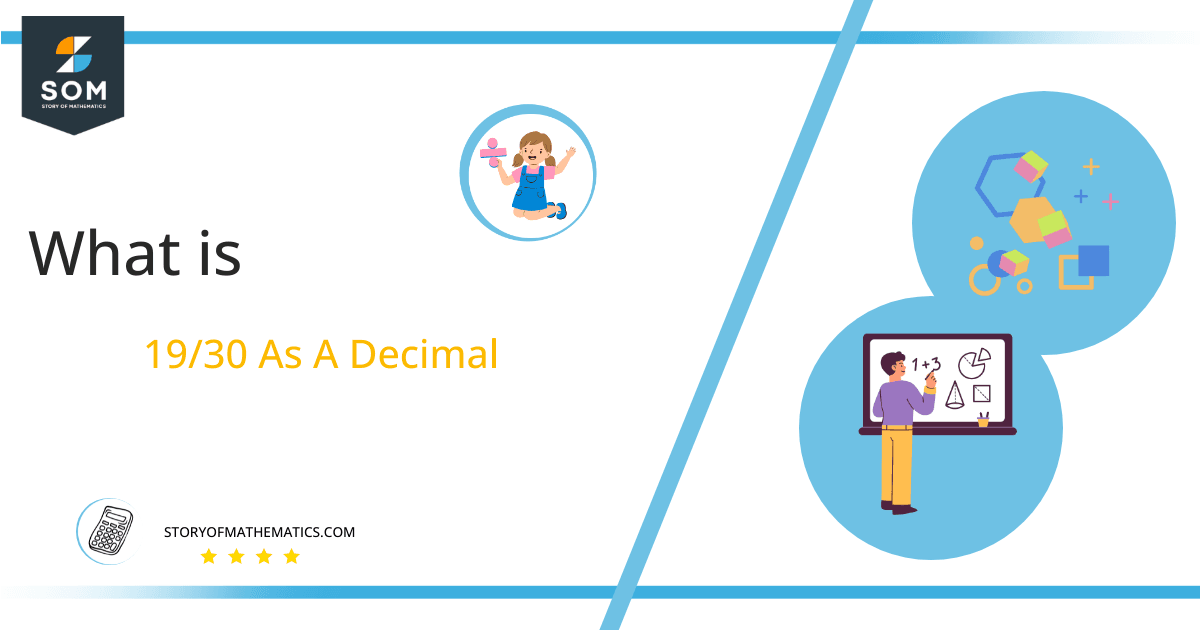# What Is 19/30 as a Decimal + Solution With Free Steps

The fraction 19/30 as a decimal is equal to 0.633.

Long division in arithmetic is a division used to divide large numbers into numerous smaller parts. A Dividend is divided by a divisor,  while the quotient shows the possible groups that can be made, and the remainder depicts how many numbers will be left undivided.

Here, we are more interested in the division types that result in a Decimal value, as this can be expressed as a Fraction. We see fractions as a way of showing two numbers having the operation of Division between them that result in a value that lies between two Integers.Now, we introduce the method used to solve said fraction to decimal conversion, called Long Division, which we will discuss in detail moving forward. So, let’s go through the Solution of fraction 19/30.

## Solution

First, we convert the fraction components, i.e., the numerator and the denominator, and transform them into the division constituents, i.e., the Dividend and the Divisor, respectively.

This can be seen done as follows:

Dividend = 19

Divisor = 30

Now, we introduce the most important quantity in our division process: the Quotient. The value represents the Solution to our division and can be expressed as having the following relationship with the Division constituents:

Quotient = Dividend $\div$ Divisor = 19 $\div$ 30

This is when we go through the Long Division solution to our problem.Figure 1

## 19/30 Long Division Method

We start solving a problem using the Long Division Method by first taking apart the division’s components and comparing them. As we have 19 and 30, we can see how 19 is Smaller than 30, and to solve this division, we require that 19 be Bigger than 30.

This is done by multiplying the dividend by 10 and checking whether it is bigger than the divisor or not. If so, we calculate the Multiple of the divisor closest to the dividend and subtract it from the Dividend. This produces the Remainder, which we then use as the dividend later.

Now, we begin solving for our dividend x, which after getting multiplied by 10 becomes 190.

We take this 190 and divide it by 30; this can be seen done as follows:

190 $\div$ 30 $\approx$ 6

Where:

30 x 6 = 180

This will lead to the generation of a Remainder equal to 190 – 180= 10. Now this means we have to repeat the process by Converting the 10 into 100 and solving for that:

100 $\div$ 30 $\approx$ 3

Where:

30 x 3 = 90

This, therefore, produces another remainder which is equal to 100 – 90 = 10. Now we must solve this problem to Third Decimal Place for accuracy, so we repeat the process with dividend 100.

100 $\div$ 30 $\approx$ 3

Where:

30 x 3 = 90

Finally, we have a Quotient generated after combining the three pieces of it as 0.633 = z, with a Remainder equal to 10.Images/mathematical drawings are created with GeoGebra.